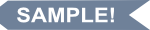# Bayesian Statistics for the Social Sciences

## Second Edition

Hardcovere-bookprint + e-book
Hardcover
November 10, 2023
ISBN 9781462553549
Price: \$69.00
250 Pages
Size: 7" x 10"
e-book
October 2, 2023
PDF ?
Price: \$69.00
250 Pages
print + e-book
Hardcover + e-Book (PDF) ?
Price: \$138.00 \$82.80
250 PagesProfessors: request an exam copy

I. Foundations

1. Probability Concepts and Bayes' Theorem

1.1 Relevant Probability Axioms

1.1.1 The Kolmogorov Axioms of Probability

1.1.2 The Rényi Axioms of Probability

1.2 Frequentist Probability

1.3 Epistemic Probability

1.3.1 Coherence and the Dutch Book

1.3.2 Calibrating Epistemic Probability Assessment

1.4 Bayes' Theorem

1.4.1 The Monty Hall Problem

1.5 Summary

2. Statistical Elements of Bayes' Theorem

2.1 Bayes' Theorem Revisited

2.2. Hierarchical Models and Pooling

2.3 The Assumption of Exchangeability

2.4 The Prior Distribution

2.4.1 Non-informative Priors

2.4.2 Jeffreys' Prior

2.4.3 Weakly Informative Priors

2.4.4 Informative Priors

2.4.5 An Aside: Cromwell's Rule

2.5 Likelihood

2.5.1 The Law of Likelihood

2.6 The Posterior Distribution

2.7 The Bayesian Central Limit Theorem and Bayesian Shrinkage

2.8 Summary

3. Common Probability Distributions and Their Priors

3.1 The Gaussian Distribution

3.1.1 Mean Unknown, Variance Known: The Gaussian Prior

3.1.2 The Uniform Distribution as a Non-informative Prior

3.1.3 Mean Known, Variance Unknown: The Inverse-Gamma Prior

3.1.4 Mean Known, Variance Unknown: The Half-Cauchy Prior

3.1.5 Jeffreys' Prior for the Gaussian Distribution

3.2 The Poisson Distribution

3.2.1 The Gamma Prior

3.2.2 Jeffreys' Prior for the Poisson Distribution

3.3 The Binomial Distribution

3.3.1 The Beta Prior

3.3.2 Jeffreys' Prior for the Binomial Distribution

3.4 The Multinomial Distribution

3.4.1 The Dirichlet Prior

3.4.2 Jeffreys' Prior for the Multinomial Distribution

3.5 The Inverse-Wishart Distribution

3.6 The LKJ Prior for Correlation Matrices

3.7 Summary

4. Obtaining and Summarizing the Posterior Distribution

4.1 Basic Ideas of Markov Chain Monte Carlo Sampling

4.2 The Random Walk Metropolis–Hastings Algorithm

4.3 The Gibbs Sampler

4.4 Hamiltonian Monte Carlo

4.4.1 No-U-Turn (NUTS) Sampler

4.5 Convergence Diagnostics

4.5.1 Trace Plots

4.5.2 Posterior Density Plots

4.5.3 Auto-Correction Plots

4.5.4 Effective Sample Size

4.5.5 Potential Scale Reduction Factor

4.5.6 Possible Error Messages When Using HMC/NUTS

4.6 Summarizing the Posterior Distribution

4.6.1 Point Estimates of the Posterior Distribution

4.6.2 Interval Summaries of the Posterior Distribution

4.7 Introduction to Stan and Example

4.8 An Alternative Algorithm: Variational Bayes

4.8.1 Evidence Lower Bound (ELBO)

4.8.2 Variational Bayes Diagnostics

4.9 Summary

II. Bayesian Model Building

5. Bayesian Linear and Generalized Models

5.1 The Bayesian Linear Regression Model

5.1.1 Non-informative Priors in the Linear Regression Model

5.2 Bayesian Generalized Linear Models

5.3 Bayesian Logistic Regression

5.4 Bayesian Multinomial Regression

5.5 Bayesian Poisson Regression

5.6 Bayesian Negative Binomial Regression

5.7 Summary

6. Model Evaluation and Comparison

6.1 The Classical Approach to Hypothesis Testing and Its Limitations

6.2 Model Assessment

6.2.1 Prior Predictive Checking

6.2.2 Posterior Predictive Checking

6.3 Model Comparison

6.3.1 Bayes Factors

6.3.2 The Deviance Information Criterion (DIC)

6.3.3 Widely Applicable Information Criterion (WAIC)

6.3.4 Leave-One-Out Cross-Validation

6.3.5 A Comparison of WAIC and LOO

6.4 Summary

7. Bayesian Multilevel Modeling

7.1 Revisiting Exchangeability

7.2 Bayesian Random Effects Analysis of Variance

7.3 Bayesian Intercepts as Outcomes Model

7.4 Bayesian Intercepts and Slopes as Outcomes Model

7.5 Summary

8. Bayesian Latent Variable Modeling

8.1 Bayesian Estimation for the CFA

8.1.1 Priors for CFA Model Parameters

8.2 Bayesian Latent Class Analysis

8.2.1 The Problem of Label-Switching and a Possible Solution

8.2.2 Comparison of VB to the EM Algorithm

8.3 Summary

9. Missing Data From a Bayesian Perspective

9.1 A Nomenclature for Missing Data

9.2 Ad Hoc Deletion Methods for Handling Missing Data

9.2.1 Listwise Deletion

9.2.2 Pairwise Deletion

9.3 Single Imputation Methods

9.3.1 Mean Imputation

9.3.2 Regression Imputation

9.3.3 Stochastic Regression Imputation

9.3.4 Hot Deck Imputation

9.3.5 Predictive Mean Matching

9.4 Bayesian Methods for Multiple Imputation

9.4.1 Data Augmentation

9.4.2 Chained Equations

9.4.3 EM Bootstrap: A Hybrid Bayesian/Frequentist Methods

9.4.4 Bayesian Bootstrap Predictive Mean Matching

9.4.5 Accounting for Imputation Model Uncertainty

9.5 Summary

10. Bayesian Variable Selection and Sparsity10.1 Introduction

10.2 The Ridge Prior

10.3 The Lasso Prior

10.4 The Horseshoe Prior

10.5 Regularized Horseshoe Prior

10.6 Comparison of Regularization Methods

10.6.1 An Aside: The Spike-and-Slab Prior

10.7 Summary

11. Model Uncertainty

11.1 Introduction

11.2 Elements of Predictive Modeling

11.2.1 Fixing Notation and Concepts

11.2.2 Utility Functions for Evaluating Predictions

11.3 Bayesian Model Averaging

11.3.1 Statistical Specification of BMA

11.3.2 Computational Considerations

11.3.3 Markov Chain Monte Carlo Model Composition

11.3.4 Parameter and Model Priors

11.3.5 Evaluating BMA Results: Revisiting Scoring Rules

11.4 True Models, Belief Models, and M-Frameworks

11.4.1 Model Averaging in the M-Closed Framework

11.4.2 Model Averaging in the M-Complete Framework

11.4.3 Model Averaging in the M-Open Framework

11.5 Bayesian Stacking

11.5.1 Choice of Stacking Weights

11.6 Summary

12. Closing Thoughts

12.1 A Bayesian Workflow for the Social Sciences

12.2.1 Coherence

12.2.2 Conditioning on Observed Data

12.2.3 Quantifying Evidence

12.2.4 Validity

12.2.5 Flexibility in Handling Complex Data Structures

12.2.6 Formally Quantifying Uncertainty

List of Abbreviations and Acronyms

References

Author Index

Subject Index